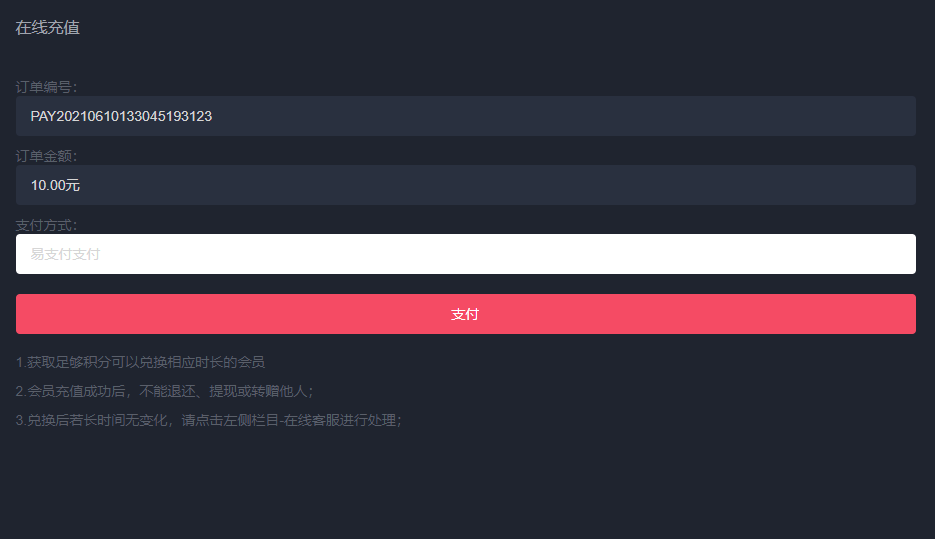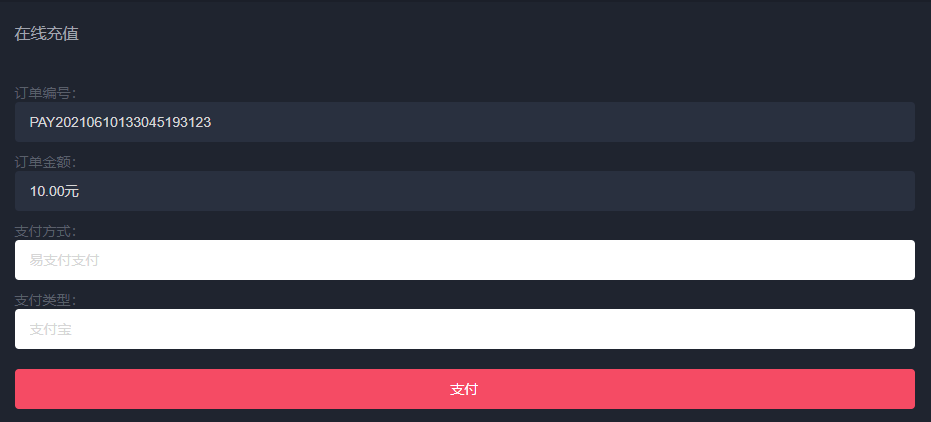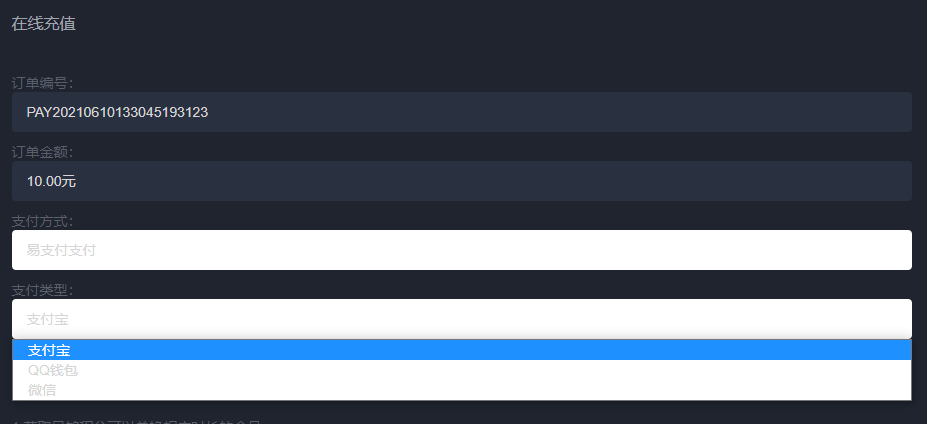`````` \$ext_list=[];
foreach(\$GLOBALS['config']['pay'] as \$k=>\$v){
if(!in_array(\$k,['min','scale','card'])){
if(!empty(current(\$v))){
\$cp = 'app\\common\\extend\\pay\\' . ucfirst(\$k);
if (class_exists(\$cp)) {
\$c = new \$cp;
\$ext_list[\$k] = \$c->name;
}
}
}
}
\$this->assign('ext_list',\$ext_list);
``````